Related Tags

control
flow
python
community creator

# What are control flow statements in Python?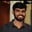Buchiredddypalli Koushik

A program’s control flow is the order in which the program’s code executes.

The control flow of a Python program is regulated by conditional statements, loops, and function calls.

Python has three types of control structures:

• Sequential - default mode
• Selection - used for decisions and branching
• Repetition - used for looping, i.e., repeating a piece of code multiple times.

## 1. Sequential

Sequential statements are a set of statements whose execution process happens in a sequence. The problem with sequential statements is that if the logic has broken in any one of the lines, then the complete source code execution will break.

## This is a Sequential statement

a=20
b=10
c=a-b
print("Subtraction is : ",c)

## 2. Selection/Decision control statements

In Python, the selection statements are also known as Decision control statements or branching statements.

The selection statement allows a program to test several conditions and execute instructions based on which condition is true.

Some Decision Control Statements are:

• Simple if
• if-else
• nested if
• if-elif-else

Simple if: If statements are control flow statements that help us to run a particular code, but only when a certain condition is met or satisfied. A simple if only has one condition to check.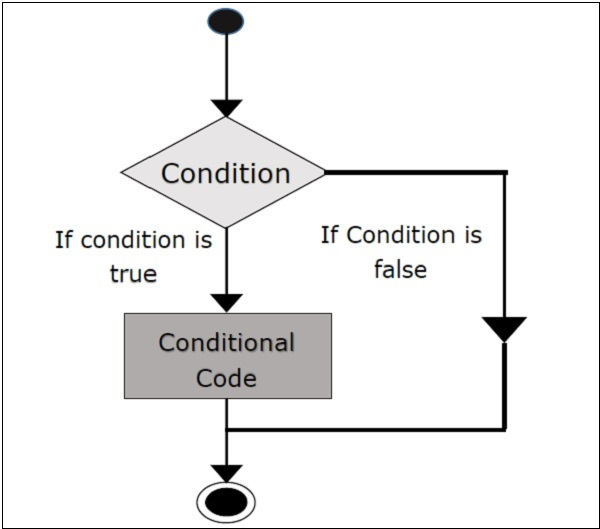n = 10
if n % 2 == 0:
print("n is an even number")

if-else: The if-else statement evaluates the condition and will execute the body of if if the test condition is True, but if the condition is False, then the body of else is executed.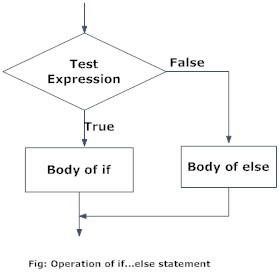n = 5
if n % 2 == 0:
print("n is even")
else:
print("n is odd")

nested if: Nested if statements are an if statement inside another if statement.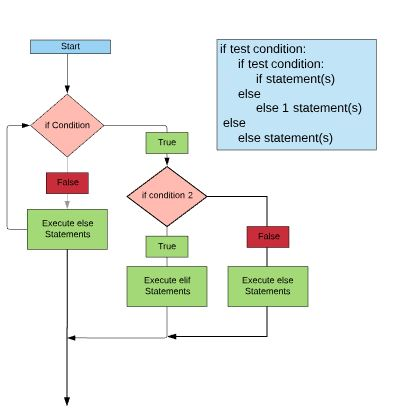a = 5
b = 10
c = 15
if a > b:
if a > c:
print("a value is big")
else:
print("c value is big")
elif b > c:
print("b value is big")
else:
print("c is big")

if-elif-else: The if-elif-else statement is used to conditionally execute a statement or a block of statements.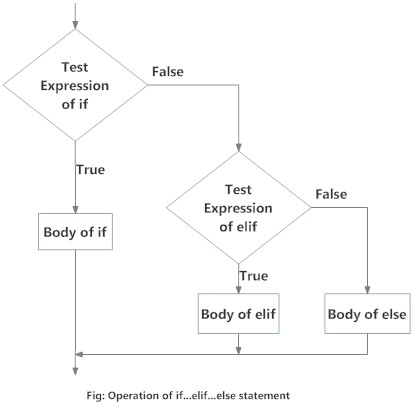x = 15
y = 12
if x == y:
print("Both are Equal")
elif x > y:
print("x is greater than y")
else:
print("x is smaller than y")

## 3. Repetition

A repetition statement is used to repeat a group(block) of programming instructions.

In Python, we generally have two loops/repetitive statements:

• for loop
• while loop

for loop: A for loop is used to iterate over a sequence that is either a list, tuple, dictionary, or a set. We can execute a set of statements once for each item in a list, tuple, or dictionary.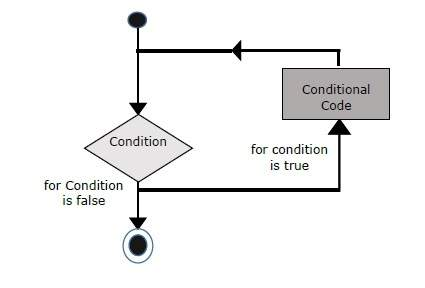lst = [1, 2, 3, 4, 5]
for i in range(len(lst)):
print(lst[i], end = " ")

for j in range(0,10):
print(j, end = " ")

while loop: In Python, while loops are used to execute a block of statements repeatedly until a given condition is satisfied. Then, the expression is checked again and, if it is still true, the body is executed again. This continues until the expression becomes false.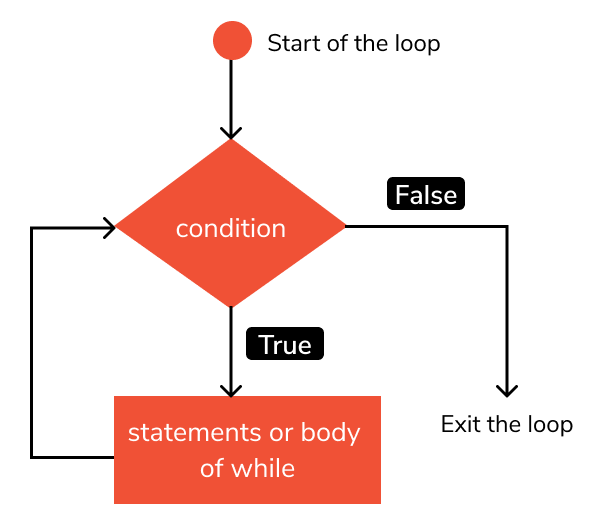m = 5
i = 0
while i < m:
print(i, end = " ")
i = i + 1
print("End")

RELATED TAGS

control
flow
python
community creator

CONTRIBUTORBuchiredddypalli Koushik
RELATED COURSES

View all Courses

Keep Exploring

Learn in-demand tech skills in half the time